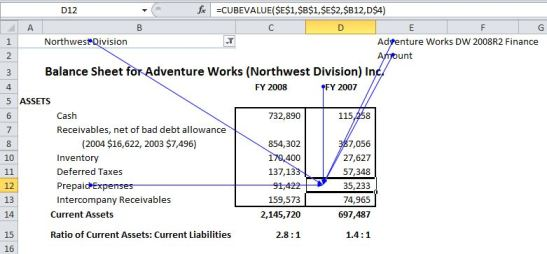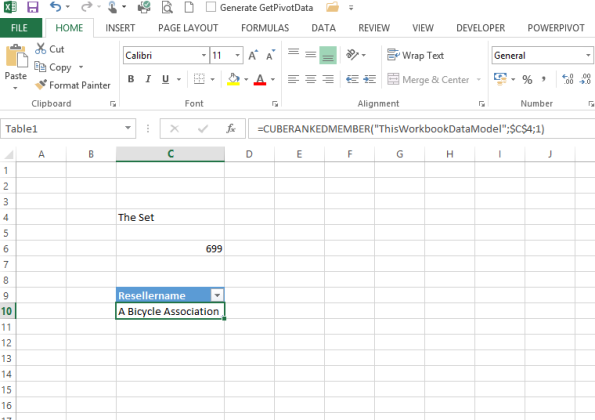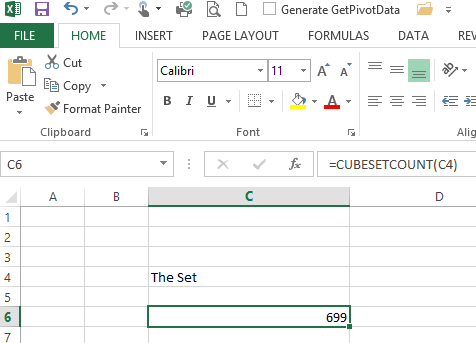#### How to Use Cube Functions in Excel

Excel provides several cube functions that you can use to aggregate and analyze data stored in OLAP (Online Analytical Processing) cubes, which are multi-dimensional data structures used for data analysis. Cube functions allow Excel to access data from an Analysis Services data source without using a Pivot Table.

There may be times when you cannot use PivotTables to submit reports directly. It is also possible that you will have to fill in the data in the format provided by your company. In this case, you can use the cube function.

There are many different cube functions:

## CUBEVALUE function

CubeValue function returns an aggregated value from the cube based on a set of members or tuples.

### The syntax of the CUBEVALUE function

=CUBEVALUE(connection, member_expression1, member_expression2…)

connection is a text string of the name of the connection to the cube

member_expression is a text string that evaluates to a member within the cube

“Value expression” is the expression that defines the value you want to retrieve, and “Set expression” is the expression that defines the set of members or tuples you want to retrieve the value for.

Here’s an example of how to use the CUBEVALUE function in Excel:

1. Connect to an OLAP cube: Before you can use the CUBEVALUE function, you must have an OLAP cube connected to your workbook.
2. Determine the value you want to retrieve: Identify the value you want to retrieve from the cube, and specify it in a value expression.
3. Determine the set of members or tuples: Determine the set of members or tuples for which you want to retrieve the value, and specify it in a set expression.
4. Enter the CUBEVALUE formula: In a blank cell, enter the CUBEVALUE formula, using the value expression and set expression you identified in steps 2 and 3 as arguments.
5. Evaluate the formula: Press the Enter key to evaluate the formula and retrieve the value from the cube based on the specified set of members or tuples.

Example formula:

=CUBEVAULE (“Sales”,” [Measures].[Profit]”, “[Time].”,” [All Product].[Beverages]”)

=CUBEVALUE (\$A\$1,” [Member].[Profit]”, D212,\$A23)The CUBEVALUE function allows you to retrieve a specific value from an OLAP cube, providing you with greater control and flexibility in analyzing your data.

## CUBEMEMBER function

CUBEMEMBER returns an element or a tuple from the cube.

### The syntax of CUBEMEMBER function

=CUBEMEMBER (connection, member_expression, )

Connection is a text string of the name of the connection to the cube

member_expression is a text string that evaluates to a member within the cube

Caption is a text string that is displayed in the cell instead of the caption. If defined, it is displayed from the cube.

Here’s an example of how to use the CUBEMEMBER function in Excel:

1. Connect to an OLAP cube: Before you can use the CUBEMEMBER function, you must have an OLAP cube connected to your workbook.
2. Identify the member you want to retrieve: Determine the name of the member you want to retrieve from the cube.
3. Enter the CUBEMEMBER formula: In a blank cell, enter the CUBEMEMBER formula, using the name of the member you identified in step 2 as the argument.
4. Evaluate the formula: Press the Enter key to evaluate the formula and retrieve the member from the cube.

Example formula:

=CUBEMEMBER (“Sales”, [months].[september])

The CUBEMEMBER function returns the specified member from the cube, allowing you to easily reference and analyze specific members within your data.

## CUBEKPIMEMBER function

The CUBEKPIMEMBER function in Excel allows you to retrieve a key performance indicator (KPI) from an OLAP cube. CUBEKPIMEMBER returns a key performance indicator (KPI) property and displays the KPI name in the cell.

### The syntax of CUBEKPIMEMBER

=CUBEKPIMEMBER (connection, kpi_name, kpi_property, )

Connection is a text string of the name of the connection to the cube

Kpi_name is a text string of the name of the kpi in the cube

Kpi_property is the Kpi component returned

• When the value is 1, the enumeration constant is “KPIValue”, which represents the actual value.
• When the value is 2, the enumeration constant is “KPIGoal”, indicating the target value.
• When the value is 3, the enumeration constant is “KPIStatus”, which indicates the status of the KPI at a specific moment.
• When the value is 4, the enumeration constant is “KPITrend”, which represents the measure of the trend value.
• When the value is 5, the enumeration constant is “KPIWeight”, which indicates the relative weight assigned to the KPI.
• When the value is 6, the enumeration constant is “KPICurrentTimeMember”, which indicates the temporary content of KPI.

Here’s an example of how to use the CUBEKPIMEMBER function in Excel:

1. Connect to an OLAP cube: Before you can use the CUBEKPIMEMBER function, you must have an OLAP cube connected to your workbook.
2. Identify the KPI you want to retrieve: Determine the name of the KPI you want to retrieve from the cube.
3. Enter the CUBEKPIMEMBER formula: In a blank cell, enter the CUBEKPIMEMBER formula, using the name of the KPI you identified in step 2 as the argument.
4. Evaluate the formula: Press the Enter key to evaluate the formula and retrieve the KPI from the cube.

Example formula:

=CUBEKPIMEMBER (“Sales”,”MySalesKPI”, 1)

=CUBEKPIMEMBER (“Sales”,”MySalesKPI”, KPIGoal,”Sales KPI Goal”)

The CUBEKPIMEMBER function returns the value of the specified KPI, allowing you to analyze and track the performance of key metrics within your data.

## CUBERANKEDMEMBER function

Returns the nth, or ranked, member in a set.

### CUBERANKEDMEMBER function syntax

=CUBERANKEDMEMBER(connection, set_expression, rank, )

Connection is a text string of the name of the connection to the cube

Set_expression the text string of the set expression.

Rank is an integer used to determine the highest value to return. If the value of this parameter is 1, the highest value is returned. If the value is 2, the second highest value is returned, and so on.

Caption is optional. It is a string of text to display in the cube cells.

“Set expression” is the expression that defines the set of members you want to retrieve from the cube, and “Rank” is the position of the member you want to retrieve within the ordered set.

Here’s an example of how to use the CUBERANKEDMEMBER function in Excel:

1. Connect to an OLAP cube: Before you can use the CUBERANKEDMEMBER function, you must have an OLAP cube connected to your workbook.
2. Determine the set of members: Identify the set of members you want to retrieve from the cube, and specify it in a set expression.
3. Determine the rank: Determine the position of the member you want to retrieve within the ordered set of members.
4. Enter the CUBERANKEDMEMBER formula: In a blank cell, enter the CUBERANKEDMEMBER formula, using the set expression and rank you identified in steps 2 and 3 as arguments.
5. Evaluate the formula: Press the Enter key to evaluate the formula and retrieve the nth member from the ordered set of members in the cube.

Example formula:

=CUBERANKEDMEMBER(“Sales”,\$D\$4,1,”Top Month”)

=CUBERANKEDMEMBER(“Sales”,CUBESET(“Sales”,”Summer”,”.[June]”,”.[July]”,”.August]”),3,”Top Month”)The CUBERANKEDMEMBER function allows you to easily retrieve members from an ordered set within an OLAP cube, providing you with greater control and flexibility in analyzing your data.

## CUBESET function

Defines a calculated set of members or tuples by sending a set expression to the cube on the server. This creates the set, and returns that set to Microsoft Excel.

### Syntax of CUBESET function

CUBESET(connection, set_expression, , [sort_order], [sort_by])

Set_expression is A text string of a set expression that results in a set of members or tuples.

Caption is a text string that is displayed in the cell instead of the caption. If one is defined, it is displayed from the cube.

Sort_order is the type of sort, if any, to perform.

Here is an example of how to use the CUBESET function in Excel:

1. Connect to the cube that contains the data you want to use.
2. In an Excel cell, type the following formula: =CUBESET(“CubeName”, “[Dimension1].[Member1],[Dimension2].[Member2]”)
3. Replace “CubeName” with the name of the cube you’re using, and replace the members with the actual members you want to include in the set.
4. Press Enter to see the results.

Example formula:

=CUBESET(“Finance”,”Order([Product].[Product].[Product Category].Members,[Measures].[UnitSales],ASC)”,”Products”)

=CUBESET(“Sales”,”Filter([Product].[Level_1].[Level_1].Members, Right([Product].[Level_1].CurrentMember.Name, 2)=””_Wisconsin””)”)

=CUBESET(“ThisWorkbookDataModel”,(A1,A2),”Product1 & Product2″)

The set expression must be written in the proper syntax, which may vary depending on the cube provider. You may need to consult the cube provider’s documentation for more information.

## CUBESETCOUNT function

Returns the number of items in a set.

### The syntax of the CUBESETCOUNT function

=CUBESETCOUNT(set)

Where Set is a text string of a Microsoft Excel expression that evaluates to a set defined by the CUBESET function.

Here is an example of how to use the CUBESETCOUNT function in Excel:

1. Connect to the cube that contains the data you want to use.
2. Create a set using the CUBESET function: =CUBESET(“CubeName”, “[Dimension1].[Member1],[Dimension2].[Member2]”)
3. In another cell, type the following formula: =CUBESETCOUNT(A1)
4. Replace “A1” with the cell reference that contains the set created in step 2.
5. Press Enter to see the results.

Example formula:

=CUBESETCOUNT (A3)